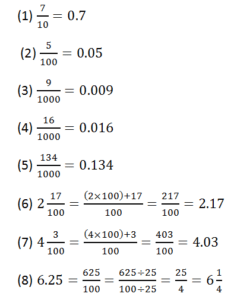# RS Aggarwal Class 6 Math Seventh Chapter Decimals Exercise 7E Solution

## RS Aggarwal Class 6 Math Seventh Chapter Decimals Exercise 7E Solution

OBJECTIVE QUESTIONS

Mark (√) against the correct answer in each of the following:(18) Which of the following is the correct order?

Ans: 2.002<2.02<2.2<2.222

(19) Which is larger: 2.1 or 2.055 = 2.1(25) 0.4 + 0.004 + 4.4

Solution: Converting the given decimals into like decimals, we get 0.400, 0.004, 4.400

So, (0.400 + 0.004 + 4.400) = 4.804

(26) 3.5 + 4.05 – 6.005

= 3.500 + 4.050 – 6.005    [converting into like decimals]

= 7.550 – 6.005 = 1.545

(27) 6.3 – 2.8 = 3.5

(28) 5.01 – 3.6

= 5.01 – 3.60 [converting into like decimals]

= 1.41

(29) 2 – 0.7

= 2.0 – 0.7   [converting into like decimals]

= 1.3

(30) 1.1 – 0.3 = 0.8Updated: May 30, 2022 — 12:24 pm